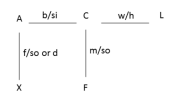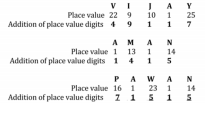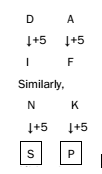# RRB ALP 2018 Practice Test Papers | Reasoning Questions (Day-18)

Dear Aspirants, Here we have given the Important RRB ALP & Technicians Exam 2018 Practice Test Papers. Candidates those who are preparing for RRB ALP 2018 can practice these Reasoning Questions to get more confidence to Crack RRB 2018 Examination.

[WpProQuiz 1698]

### Click “Start Quiz” to attend these Questions and view Solutions

1. A is the brother of C and the father of X. F is the son of C and C is the wife of L. What is the relationship of F to X?
1. Mother
2. Sister
3. Brother
4. Cousin
1. ZYXWVU, YXWVUZ,?, WVUZYX, VUZYXW
1. XWVUZY
2. XWVUYZ
3. XWUVZY
4. XVWUZY
1. In a certain code, ‘VIJAY’ is written as ‘49117’ and ‘AMAN’ is written as ‘1415’. How is ‘PAWAN’ written in the same code?
1. 71155
2. 71515
3. 17515
4. 71551
1. If ‘+’ means ‘divided by’, ‘–’ means ‘added to’, ‘*’ means ‘subtracted from’, and ‘/’ means ‘multiplied by’, then 26 – 15 + 5 × 4/ 2 =?
1. 15
2. 20
3. 25
4. None of the above
1. If ‘DA’ is related to ‘IF’, ‘NK’ will be related to
1. SP
2. PS
3. PR
4. SR
1. Insert the missing number. 0, 4, 18, 48, 100, ___
1. 132
2. 150
3. 162
4. 180
1. Which of the following is different from the others?
1. AF
2. JN
3. LQ
4. WB
1. Man: Lip:: Bird:?
1. Bush
2. Grass
3. Forest
4. Beak
1. JKP, KLQ, LMR, MNS,?
1. NOT
2. NPT
3. PNS
4. MPS
1. Ornithologist: Bird:: Archaeologist:?
1. Islands
2. Mediators
3. Archaeology
4. Aquatic

Solution:Where, b – brother, si – sister, so – son, d – daughter, w – wife and h – husband Clearly F is the cousin of X.

In the given series, the next term is found by moving the first letter at the end of the term. The required term is XWVUZY.

The logic used here is given as follows:If we put with correct symbols, the equation “26 – 15 + 5 * 4/ 2” would be written as “26 + 15/ 5 – 4 × 2”. = 26 + 3 – 4 × 2 = 26 + 3 – 8 = 21The pattern of the series is as follows:

13 – 12 = 0

23 – 22 = 4

33 – 32 = 18

43 – 42 = 48

53 – 52 = 100

The next number = 63 – 62 = 180

By following the English alphabet letter position, the second letter is +5 from the first letter in all the options, except ‘JN’.

Lip is a human body part while the beak is a bird body part.

In the given series, letters in each term consist of their predecessor. Hence, the required term is (M + 1) (N + 1) (S + 1), i.e. NOT.

An ‘ornithologist’ studies about ‘birds’. Similarly, an ‘archaeologist’ is involved with the study of ‘archaeology’.

RRB ALP 2018 Practice Test Papers | Reasoning Questions (Day-1)

RRB ALP 2018 Practice Test Papers | Reasoning Questions (Day-2)

RRB ALP 2018 Practice Test Papers | Reasoning Questions (Day-3)

RRB ALP 2018 Practice Test Papers | Reasoning Questions (Day-4)

RRB ALP 2018 Practice Test Papers | Reasoning Questions (Day-5)

RRB ALP 2018 Practice Test Papers | Reasoning Questions (Day-6)

RRB ALP 2018 Practice Test Papers | Reasoning Questions (Day-7)

RRB ALP 2018 Practice Test Papers | Reasoning Questions (Day-8)

RRB ALP 2018 Practice Test Papers | Reasoning Questions (Day-9)

RRB ALP 2018 Practice Test Papers | Reasoning Questions (Day-10)

RRB ALP 2018 Practice Test Papers | Reasoning Questions (Day-11)

RRB ALP 2018 Practice Test Papers | Reasoning Questions (Day-12)

RRB ALP 2018 Practice Test Papers | Reasoning Questions (Day-13)

RRB ALP 2018 Practice Test Papers | Reasoning Questions (Day-14)

RRB ALP 2018 Practice Test Papers | Reasoning Questions (Day-15)

RRB ALP 2018 Practice Test Papers | Reasoning Questions (Day-16)

RRB ALP 2018 Practice Test Papers | Reasoning Questions (Day-17)